# 附录、线性模型和线性代数基

## 线性分类概述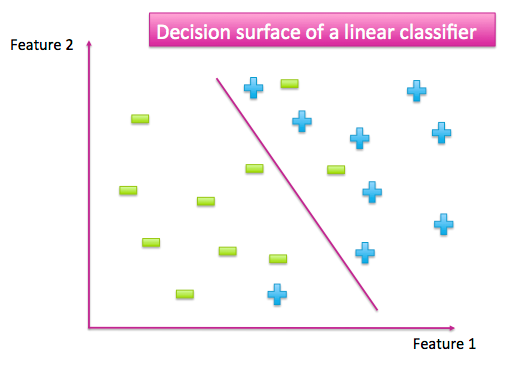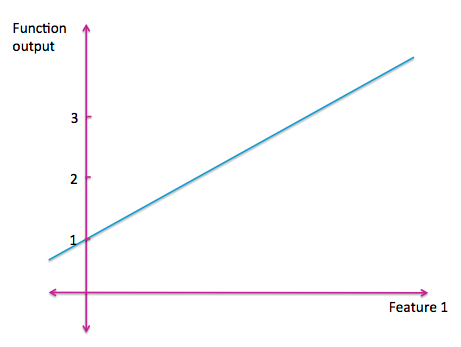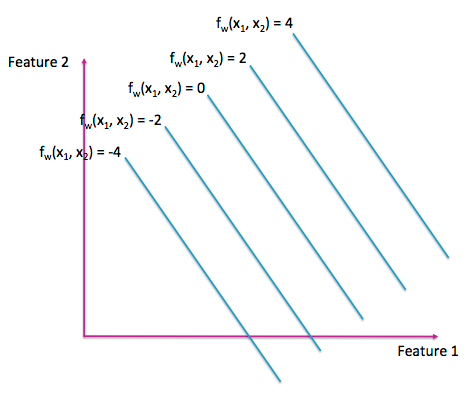## 矩阵的解析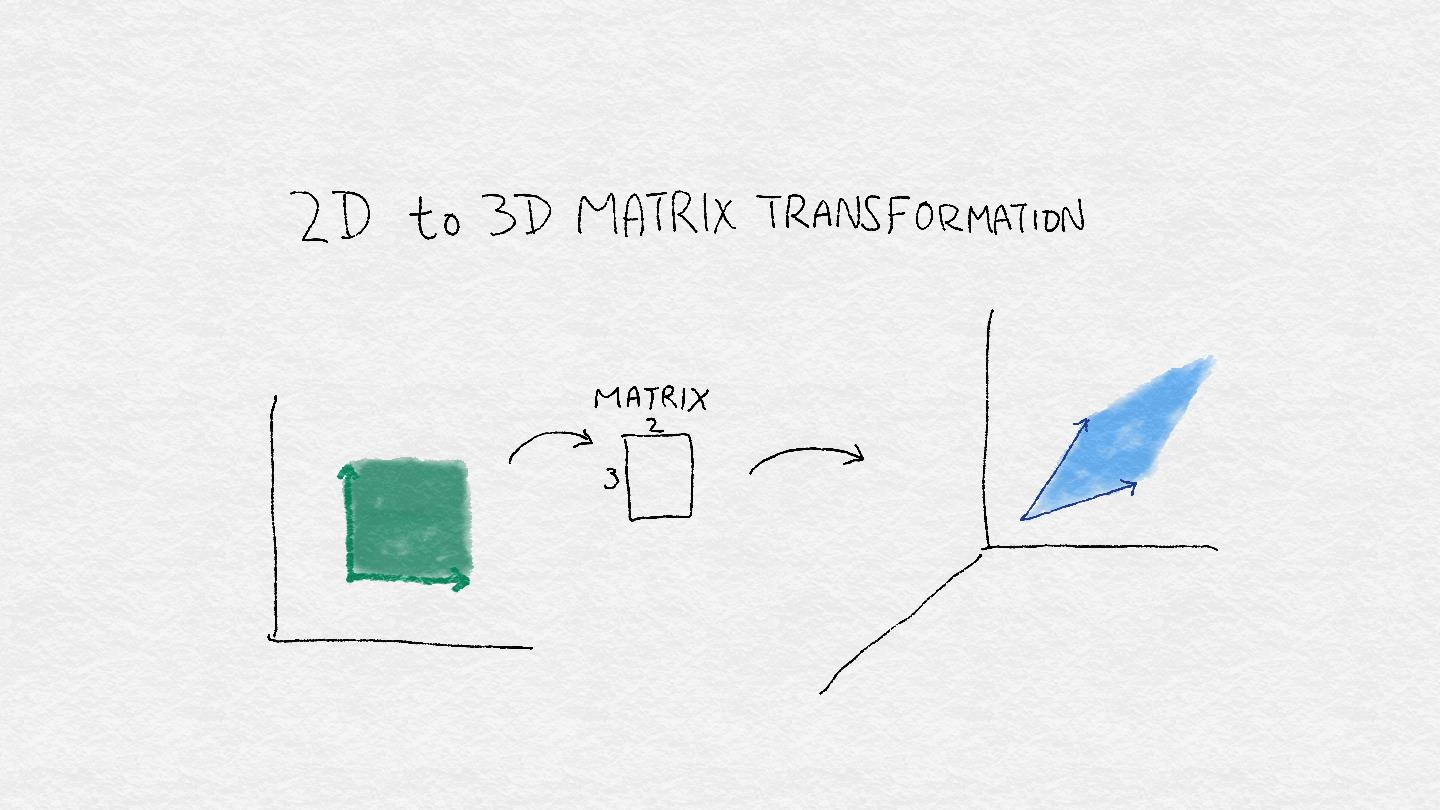## 从矢量到子空间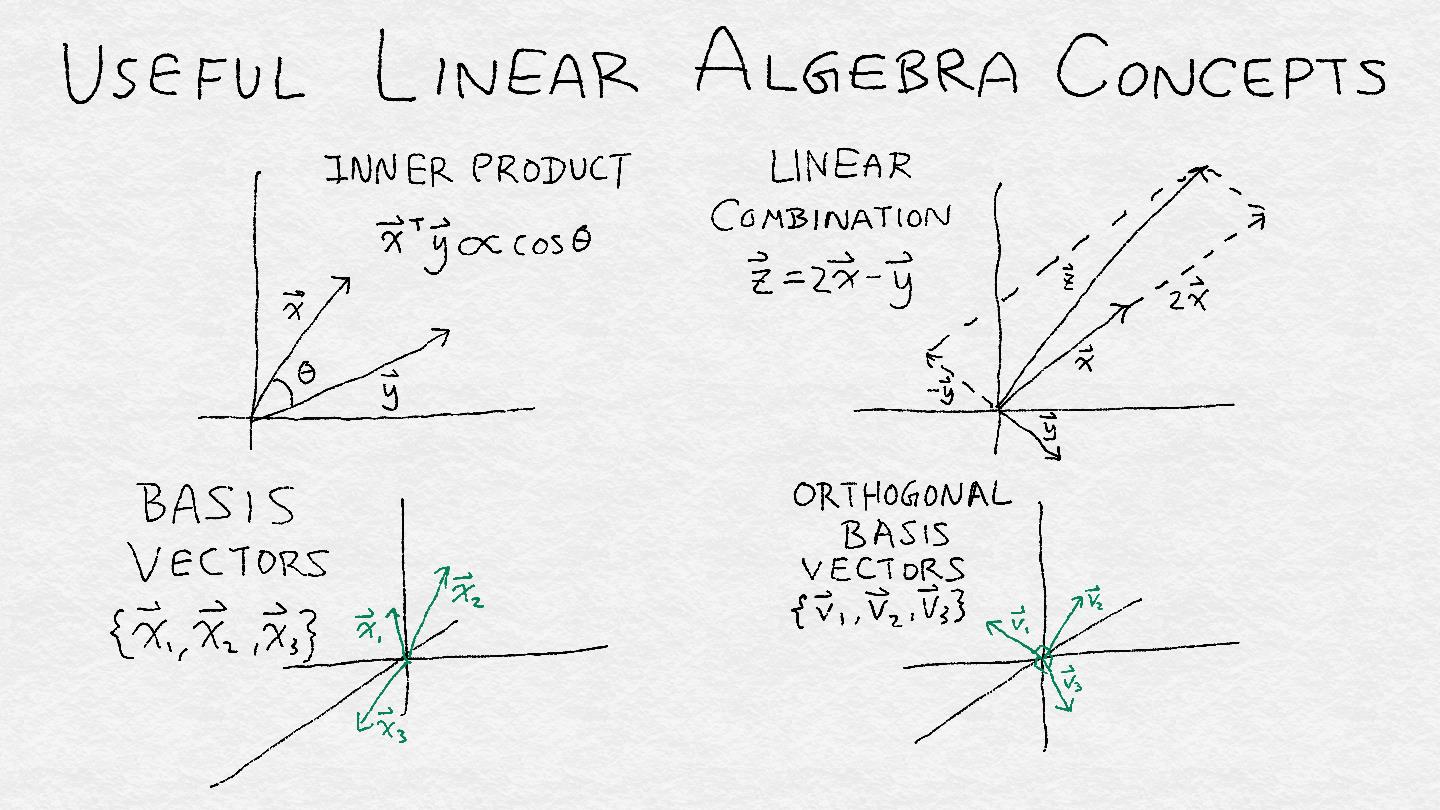#### 有用的线性代数定义

• 标量：一个数c，不是矢量
• 向量：$$x=(x_1,x_2,…,x_n)$$
• 线性组合：$$ax+by=ax_1+by_1,ax_2+by_2,…,ax_n+by_n$$
• 向量组的范围：向量组 $$u=a_1 v_1+⋯+a_k v_k$$$$a_1,…,a_k$$ 任意
• 线性独立性：xy相互独立，如果x≠cyc是任何标量常量
• 内在产品：$$x,y=x_1 y_1+x_2 y_2+⋯+x_n y_n$$
• 正交矢量：如果x,y=0，两个向量x,y正交
• 子空间：包含更大的矢量空间中的矢量子集，满足这三个标准：
• 包含 0 向量
• 如果包含一个向量v，那么也包含所有向量cv，其中c是一个标量。
• 如果包含两个向量uv，那么也包含u+v
• 基：一组跨向子空间的向量
• 正交基：一个基 $${v_1,v_2,…,v_d}$$$$v_i,v_j=0$$i,j任意
• 子空间的秩：跨越子空间的线性无关基向量的最小数目。

## 奇异值分解（SVD）

#### 特征向量和奇异向量

A是一个n*n的矩阵。如果这里有一个向量v和一个标量λ，使Av=λvv称作特征向量，λ称为A的一个特征值。

A成为一个长方形矩阵。如果这里有一个向量u和一个向量v和一个标量σ，那么Av=σu$$A^T u=σv$$uv被叫做左右奇异向量，σA的奇异值。

$$A=U \Sigma V^T$$

• 将输入向量映射到右奇异向量基V上
• 通过相应的奇异值缩放每个坐标
• 将此分数与每个左奇异向量相乘
• 总结结果

A是实矩阵（即所有元素都是实值）时，所有的奇异值和奇异向量都是实值的。奇异值可以是正数，负数或零。矩阵的有序奇异值集称为谱，它揭示了很多矩阵。奇异值之间的差距会影响解的稳定性，最大绝对奇异值与最小绝对奇异值之间的比值（条件数）会影响迭代求解器的求解速度。这两个属性都对可找到的解决方案的质量产生显着影响。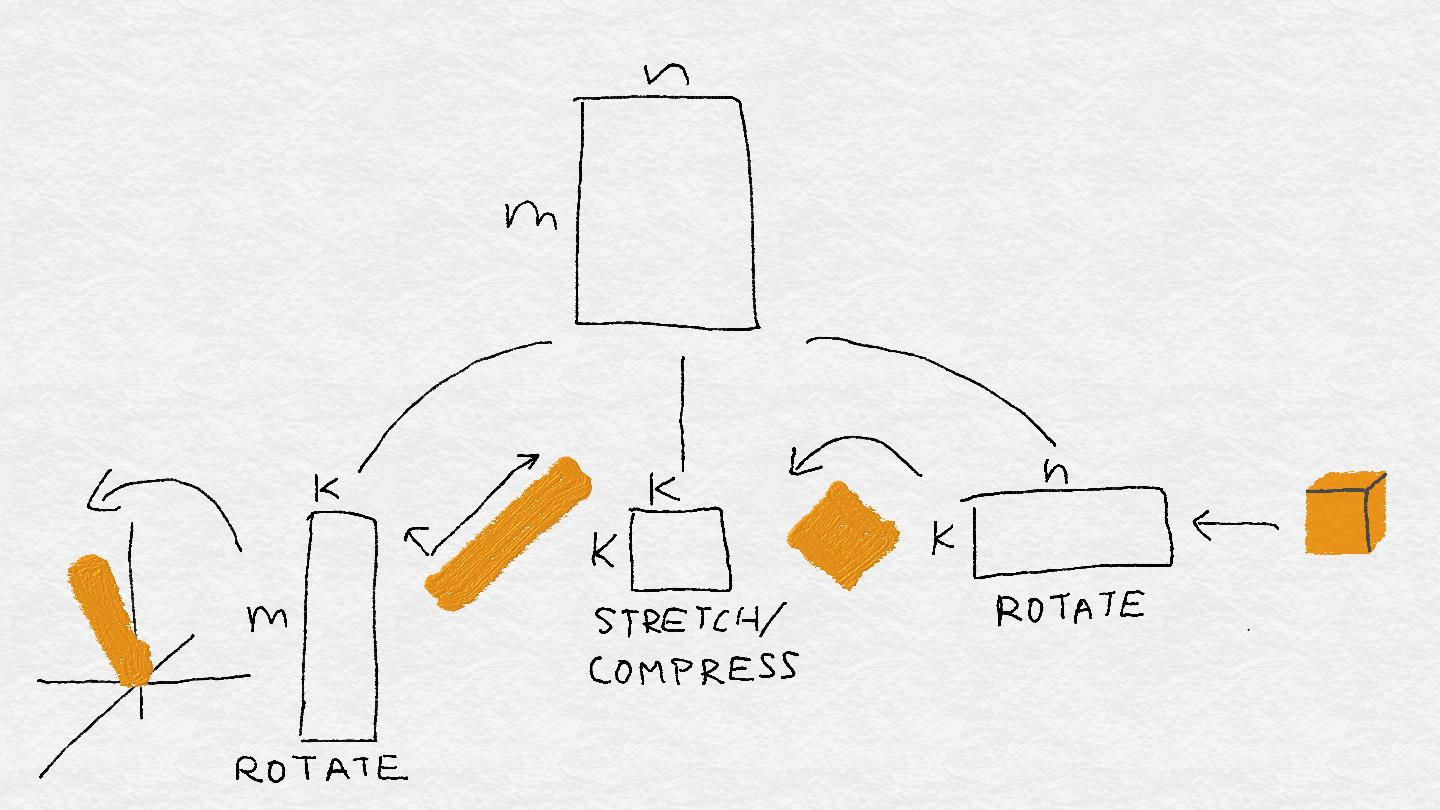## 数据矩阵的四个基本子空间

#### 数据矩阵

A：行是数据点，列是特征。

### 求解一个线性系统

A(Wparticular + Wsad-trumpet) = y


Wgeneral = Wparticular + Whomogeneous


Wparticular 是方程Aw=y的精确解，可能有也可能没有这样的解。如果没有，那么系统只能近似地解决。如果有，那么y属于已知的a的列空间，列空间是A可以映射到的向量集合，通过它的列的线性组合。

Whomogeneous 是方程Aw = 0的解。（Wsadd-trumpet 的完整名称是“Whomogeneous”。）这一点现在应该很熟悉了。所有的广义向量的集合构成了a的零空间，这是具有奇异值为 0 的右奇异向量张成的空间。

“零空间”这个名字听起来像是一场生死攸关的危机的结局。如果零空间包含除零向量之外的任何向量，那么就有方程Aw = y有无穷多个解，有太多的解可供选择

1、标签向量可以通过特征子集(列向量)的线性组合很好地逼近。更好的是，特性集应该是线性无关的。 2、为了使零空间很小，行空间必须很大。(这是因为这两个子空间是正交的。)数据点(行向量)的集合越线性无关，零空间就越小。

•为了便于求解，数据矩阵的条件数——最大奇异值与最小奇异值之比——应该很小。

## 分类器的概述

### K-最近邻

K-最近邻(kNN) Scikit Learn的sklearn-neighbors包